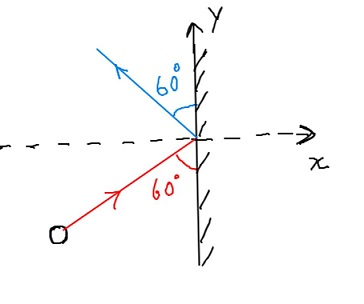# A 3.00 kg steel ball strikes a wall with a speed of 10 m/s at an angle of 60^\circ with the...

## Question:

A 3.00 kg steel ball strikes a wall with a speed of 10 m/s at an angle of {eq}60^\circ {/eq} with the surface. It bounces off with the same speed and angle. If the ball is in contact with the wall for 0.200 s, what is the average force exerted by the wall on the ball?## Newton's Second Law of Motion:

When a ball hits the wall, and rebounce back, the force exerted by the wall on the ball causes change in linear momentum of the ball, which is based on Newton's Second Law of motion.:

## Answer and Explanation:

We are given:

• A ball of mass, m = 3.00 kg
• Initial velocity of ball {eq}v_i = 10\ m/s{/eq}, making an angle {eq}\theta_i = 60^\circ{/eq} with the surface of the wall
• Final velocity of ball, {eq}v_f = 10\ m/s{/eq} , with rebounce angle {eq}\theta_f = 60^\circ{/eq} with the surface of the wall.
• Contact time of ball with the wall, {eq}\Delta t = 0.200\ s{/eq}

Initial velocity vector {eq}(\vec v_i){/eq} of the ball

• {eq}\vec v_i = v_i \sin 60^\circ \hat i + v_i \cos 60^\circ \hat j\ m/s{/eq}
• {eq}\vec v_i = 10\times \sin 60^\circ\hat i + 10\times \cos 60^\circ\hat j = 8.66\ \hat i + 5\ \hat j\ m/s{/eq}

Final velocity vector {eq}(\vec v_f){/eq} of the ball

• {eq}\vec v_f = - v_f \sin 60^\circ \hat i + v_f \cos 60^\circ \hat j{/eq}
• {eq}\vec v_f = - 10\times \sin 60^\circ\hat i + 10\times \cos 60^\circ\hat j = - 8.66\ \hat i + 5\ \hat j\ m/s{/eq}

Finding average force {eq}(\vec F){/eq} exerted by wall on the ball

Applying Newton's Second Law:

• {eq}\rm \vec F = \dfrac{change\ in\ momentum\ of\ ball}{Contact\ time\ of\ ball}{/eq}
• {eq}\vec F = \dfrac{m\times (\vec v_f - \vec v_i)}{\Delta t}{/eq}
• {eq}\vec F = \dfrac{3\times ((-8.66\ \hat i + 5\ \hat j) - (8.66\ \hat i + 5\ \hat j))}{\Delta t}{/eq}
• {eq}\vec F = \dfrac{-3\times 2\times 8.66\ \hat i}{0.2}{/eq}
• {eq}\vec F = -259.8\ N\ \hat i{/eq}

#### Learn more about this topic:Practice Applying Newton's Second Law

from Physics 111: Physics I

Chapter 6 / Lesson 9
2.5K